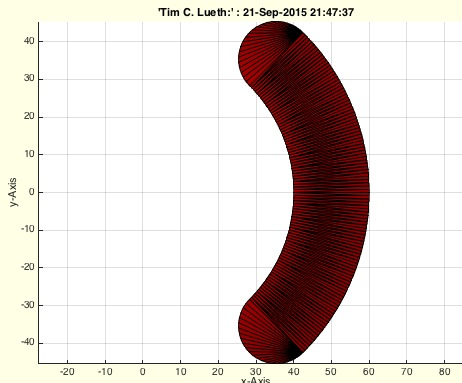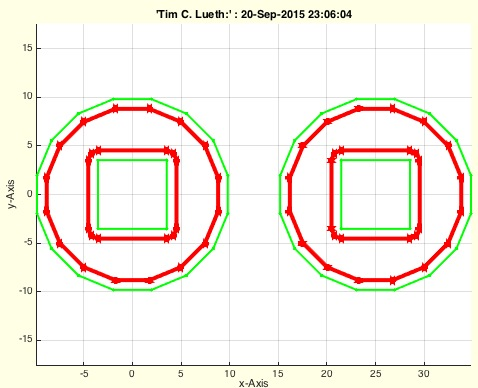% PLkidney(RI,RO,w,n) - returns a kindey shaped point list% (by Tim Lueth, VLFL-Lib, 2015-SEP-21 as class: CLOSED POLYGON LISTS)% % Tim Lueth's primitive version of Yannick Krieger's advanced kidney % fnctn (Status of: 2017-01-05)% % See also: PLcircle, PLcircseg, PLevolvente, PLgear, PLhelix, PLrand, % PLspiral, PLsquare, PLstar% % PL=PLkidney(RI,RO,[w,n])% === INPUT PARAMETERS ===% RI: Inner radius% RO: Outer Radius% w: angle of circular segment% n: number of points per element;default is '' = auto% === OUTPUT RESULTS ======% PL: Point List% % EXAMPLE: % PLkidney(40,60,pi/2,40)% PLkidney(40,60,100,40)% PLkidney(2,10,pi,10)%% CPLgrowEdge(CPL,w) - grows a contour line in a distance% (by Tim Lueth, VLFL-Lib, 2015-SEP-20 as class: CLOSED POLYGON LISTS)% % hui hui hui. One lost day work and than a solution within 30 minutes.% (Status of: 2015-09-20)% % [RPL,ENL,PNL,PDL]=CPLgrowEdge(CPL,w)% === INPUT PARAMETERS ===% CPL: Closed Polygon line% w: distance for growing% === OUTPUT RESULTS ======% RPL: Recalculated CPL% ENL: Edge normal vector list% PNL: Point normal vector lit% PDL: Point direction vector list% % EXAMPLE: % CPLgrowEdge(PLstar(10,12),1);% CPLgrowEdge(PLstar(10,12),-1);% CPLgrowEdge(flipud(PLstar(10,12)),1);% CPLgrowEdge(flipud(PLstar(10,12)),-1);%